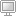#Pages:    Go Down

###AuthorTopic: Scale Degrees (In-Depth Look)  (Read 3260 times)

####T-Block

• Moderator
• LGM Member
••• Posts: 17289
• Gender:• I got my MBA!!!##### Scale Degrees (In-Depth Look)
« on: April 30, 2007, 04:10:49 PM »
Scale Degrees

By now, we should all know what a scale degree is.  This post is just a little more in-depth look at them.  Let's review what we you all should already know from my previous posts, examples will be in the key of C:

Major scale formula =   __ WS __ WS __ HS __ WS __ WS __ WS __ HS __

C major scale =  C  D  E  F  G  A  B  C

From the major scale, each note get's a number:

1 = C
2 = D
3 = E
4 = F
5 = G
6 = A
7 = B

If the scale goes past an octave, u can keep going with the numbering:

8 = C
9 = D
10 = E
11 = F
12 = G
13 = A

That's as far as the numbering goes.  All of that above should be familiar to most of you.  Here are a few things you may or may not know about the scale degrees:

Roman Numerals

In the early start of music theory, they used Roman Numerals to name the scale degrees.  So, if you go to a real theory class, you will see the scale degrees number like this:

1 = I
2 = II
3 = III
4 = IV
5 = V
6 = VI
7 = VII

Scale Degree Names

Each of the 1st seven scale degrees also has a specific name.  Let me break it down for you:

I = tonic (principle note in a key)

II = supertonic (the next note above the principle note)

III = mediant (half-way between the tonic and the dominant)

IV = subdominant (5th below tonic)

V = dominant (5th above tonic, the dominant element in a key)

VI = submediant (half-way between the dominant and the tonic)

Roman Numeral Chord Naming

Another important factor in naming these Roman Numerals is whether it's upper case or lower case.  Upper case Roman Numerals signify that the base of the chord is major.  Lower case Roman Numerals signify that the base of the chord is minor.  Example:

Major chords =  I  II  III  IV  V  VI  VII
Minor chords =  i  ii  iii  iv  v  vi  vii

The Roman Numerals stop there, no 9 = IX, etc.  These Roman numerals are used in naming chords associated with music.  These 1st seven scale degrees are the roots for all chords in a particular key.  So:

I = major chord built off the 1st scale degree
II = major chord built off the 2nd scale degree
etc.

i = minor chord built off the 1st scale degree
ii = minor chord built off the 2nd scale degree
etc.

For chords other than major or minor, you have to notate them differently:

diminished - the base of a diminished chord is minor, so it starts with a lower case roman numeral, then a small degree symbol is placed in the upper right hand corner of it.  Example:

i* (i don't know how to make a degree symbol, so i used an asterik to represent it)

augmented - the base of an augmented chord is major, so it starts with an upper case roman numeral, then a small + symbol is placed in the upper right hand corner of it.  Example:

I+

9ths, 11ths, 13ths - these chords are in a whole different league than the rest of the chords.  So, I'm just gonna give you the naming of them in dominant form.  The base of a dominant 9th, 11th, or 13th is major, so give it the appropriate Roman Numeral, then after that a small 9, 11, or 13 is placed in the upper right hand corner of it.  Example:

I9
I11
I13

I'll have to do another post of 9ths, 11ths, and 13ths as it pertains to scale degrees and chord naming as soon as I organize the information in a way I think will be understood by yall.  If anyone has any questions on this, don't be afraid to ask.

If any other theory people wanna add to this topic, feel free to do so.
Real musicians play in every key!!!
Music Theory, da numbers work!
Pages:    Go Up

LGM2 design by Bloc